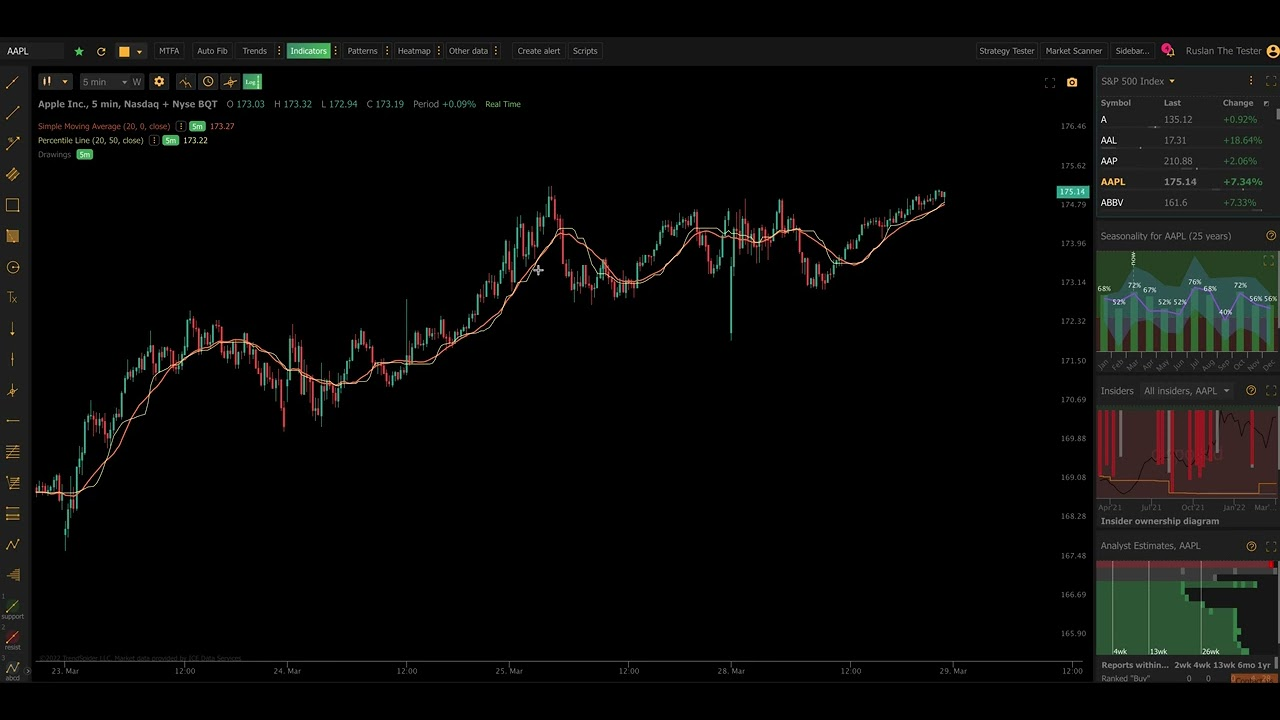03/30/2022 |

# TrendSpider Software Update: The Percentile Line Indicator

Hello Everyone, and welcome to yet another software update! In this update, we are introducing a new type of experimental indicator that our engineering team has developed called the ‘Percentile Line’. More after the jump!

## Average vs. Median

Most traders are familiar with moving averages, which plot a rolling average of a series of values over a specific window size. For example, the simple 50-period moving average is always the average of the most recent 50 numbers in a series. Moving averages have a handful of great use cases but they also have some pitfalls, such as their smoothing nature, which adds to the lag of the data coming into them. The larger the series of numbers (bigger the window size) the slower they move. Percentile lines also have a lag effect, but it is different in a number of ways.

Enter; Medians. A median number is the middle-most number in a series. Unlike a moving average, there is no addition and division. We simply take the middle-most point in the series and that becomes the median. TheBalance.com has a great article about this mathematical concept that explains it far better than we can. In other words, this means traditional moving averages are skewed by outliers by there very nature. This does not make moving averages bad, but it should be understood.

For instance, take a set of numbers: 1, 2, 3, 18, 20. The average value of these numbers is 11. The median value, however, is 3.

• The average is “sum of all the numbers divided by the amount of numbers in the set”.
• The median is “such a value that 50% of data points do not exceed it”.
• In the case that change the last number of this data set to 200 (and thus have 1, 2, 3, 18, 200), the average would become 56. The median, however, will remain 3.

Averaging produces a number that does not tell you how the base values are being distributed. Median does, and percentiles do, as well.

Median is an example of what they call “percentiles”; Median is the 50th Percentile.

## The Percentile Line Indicator

The Percentile Line indicator is a special indicator that we have developed and released into TrendSpider. We consider it experimental — meaning we believe it is useful, but we have not defined specific use cases for it yet.

This indicator allows you to set a defined window length and plot a percentile line on it. To plot the Median of price action over a rolling window, set the window to 50%.

The below chart demonstrates a 70 Period 50th Percentile Line (Black) relative to a 70 Period Simple Moving Average (Green). As you can see, the percentile line reacts to price more quickly in some cases, and slower in other cases.In the below screenshot, we show 5 Median lines that are all set to the same 50-period window:

• 0th percentile  — “the min value for the data set”
• 25th percentile
• 50th percentile — “the Median”
• 75th percentile
• 100th percentile — “the max value for the data set”As you can see, there are some interesting patterns here. For example, when the trend starts to reverse, the percentile lines seem to flatten out. When price is trending, it is very clear because of their slope and direction.

100th Percentile and 0th Percentile would have acted just like Donchian Channels, should 0th have Price Source of “low” and 100th have price source of “high”. Note how the same charts look with “length” being 150 instead of 50.### Adjusting the Sensitivity of a Percentile Line

For a simple moving average, you only have one way to adjust the sensitivity of your line: The length value. For the Percentile Line, you can manipulate with the Percentile parameter, which naturally impacts the sensitivity without affecting the degree the indicator lags. Check out the Percentile lines pictured in the chart below. All of them have the same “Length” value, but the “Percentile” values have been set to Fib numbers.## Exploring in TrendSpider Platform

Here is a video that shows off how to add it to your charts and explains how it works:## Works Across TrendSpider

This indicator works across the entire TrendSpider platform, including in scanners, alerts, smart checklists, backtests and, of course, on charts.

We hope you enjoy it. Let us know what you think and how you use it!

• Share: